# CALC Two Equation Assitance

##### Royal Flush
My boy is in Calc two and is stuck on this problem.I can barely figure out pot odds, let alone this shit (that doesn't even look real).

I told him I would post it. He didn't want me to, but I figured, fuck it.

#### Ben

##### Full House
Well, now you've depressed me. In high school/college I could do this shit like it was nothing. Today? I don't have a farking clue.

I DO still know what it means, which is a win, I guess...:

##### Royal Flush
He just texted me and said he figured it out. He's 18, will have his bachelor's degree at 19.5 ish in Actuarial Sciences. ...oh, debt free

•iblonger

#### jbutler

42?

##### Full House
My gut says it's approaching (1 + SQRT(2) ) / 2... (about 1.2071...) but I don't remember how to prove it. Or I may just be wrong.

##### Full House
No, it should be slightly more than that, because the curve from SQRT(1) to SQRT(2) bows slightly upwards...

GAH... brain hurts...

#### Mr. Cheese

##### Full House
E=mc^2

Boom! Mind Blown!

2/3

#### abby99

##### Full House
Supporting Member
'Bout tree fiddy.•Chicken Rob, Big Jilm and BGinGA

#### BGinGA

##### Royal Flush
Tourney Director
Supporting Member
'Bout tree fiddy.lolol marsha wins teh interwebz today....

•abby99

##### Full House

Unless I'm wildly mistaken, it ain't 2/3.

The expansion has n terms to sum up, each of which is 1/n (or larger). The sum of n terms of 1/n is exactly one... The sum of this series should always be greater than 1.

For n=1000, for example:

- the first term is ( SQRT (1000/1000) / 1000, which is (SQRT (1) ) / 1000, which is 1/1000, which is 0.001.
- the last term is ( SQRT(1999/1000) ) / 1000, which is (SQRT (1.999) ) / 1000, which is 1.41386/1000, which is .001413859
- all the other terms are between those two values, progressing slowly from 1 to 1.41386 (all over 1000).

As n goes to infinity, the first term stays 1/n... but the last term becomes SQRT(2)/n. So the numerator goes from 1 to SQRT 2.

The sums of the 1/n portions add up to one... the rest of it gradually rises, and as n approaches infinity, the last term approaches the square root of two...
Or, graphed, the 1/n parts (times n) form a square. The rest of the terms are the area above y=1, which gradually rises towards about 1.414, which is the square root of two.

The answer is really the shaded area under the curve f(x) = SQRT (x):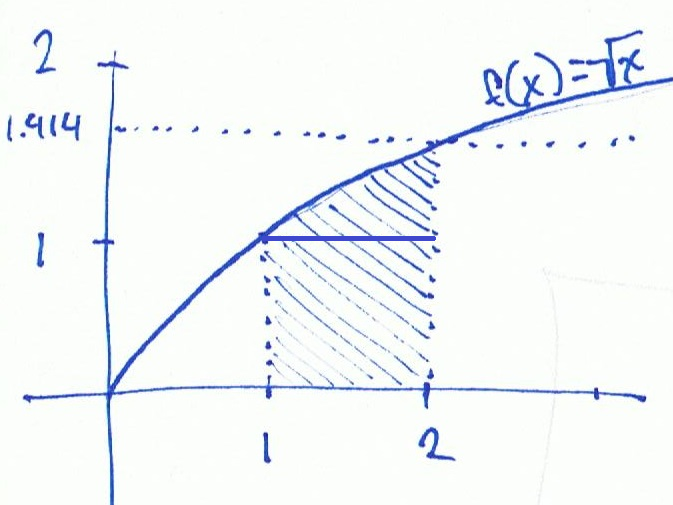Unless, of course, I've goofed. But that shaded area looks, to me, like more than 2/3. It's more than 1, it's less than 1.414... but my original answer was wrong, because you can't use an average to follow that curve - it's not a straight line from y=1 to 1=SQRT(2).

#### SixSpeedFury

##### Full House
Supporting Member
I would like to answer Alex. What is calculus?

•Big Jilm

#### spikeithard

##### Flush" looks like something they found on the ship at Roswell" haha

#### Big Jilm

##### Full House
GearX can do teh maths

##### Royal Flush
Unless I'm wildly mistaken, it ain't 2/3.

The expansion has n terms to sum up, each of which is 1/n (or larger). The sum of n terms of 1/n is exactly one... The sum of this series should always be greater than 1.

For n=1000, for example:

- the first term is ( SQRT (1000/1000) / 1000, which is (SQRT (1) ) / 1000, which is 1/1000, which is 0.001.
- the last term is ( SQRT(1999/1000) ) / 1000, which is (SQRT (1.999) ) / 1000, which is 1.41386/1000, which is .001413859
- all the other terms are between those two values, progressing slowly from 1 to 1.41386 (all over 1000).

As n goes to infinity, the first term stays 1/n... but the last term becomes SQRT(2)/n. So the numerator goes from 1 to SQRT 2.

The sums of the 1/n portions add up to one... the rest of it gradually rises, and as n approaches infinity, the last term approaches the square root of two...
Or, graphed, the 1/n parts (times n) form a square. The rest of the terms are the area above y=1, which gradually rises towards about 1.414, which is the square root of two.

The answer is really the shaded area under the curve f(x) = SQRT (x):

View attachment 11122

Unless, of course, I've goofed. But that shaded area looks, to me, like more than 2/3. It's more than 1, it's less than 1.414... but my original answer was wrong, because you can't use an average to follow that curve - it's not a straight line from y=1 to 1=SQRT(2).

the only problem I had with that was choosing the domain, for the first term, delta x, you are given 1/n which is decided by ((b-a)/n), with actual numbers that could be (1-0)/n, or from (2-1)/n, (3-2)/n, etc, would all give you the partition 1/n, where the answer 2/3 came from was by using the domain (0,1), so that would be integrating SQRT X between the x values of 0 and 1, which would come out to be 2/3 (1^3/2 - 0^3/2), but yes, with the domain being set at (1,2) you are given a rough answer of 1.2189514. So I guess my confusion is coming from why use (1,2) as your domain, as apposed to something else? So I guess I'm just not understanding where the first and last term of the series are originating from?

"my son's reply, I have no idea what any of this means".

Last edited:

##### Full House
Well, for starters, I could just be plain ol' wrong. But here's how I looked at different parts of the construction to try to make intuitive and visual sense out of the exapnsion, and what happen when you approach infinity... I'm going to walk through my logic step-by-step, so you can see how I got there (or top me right where I'm making a goof.)

First, to make sense of the 1/n part in the front, which can easily confuddle - your expansion will always have n terms to sum... and each of them will be multiplied by 1/n. So, for example, if n is ten, you have ten terms, each multiplied by 1/10th. That means this: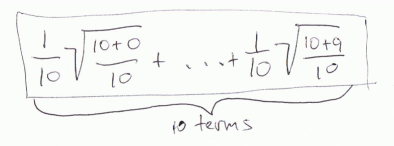It's like slicing the series into ten pieces and then adding them up. I can make better sense of what I'm "slicing up" if I move the 1/10th... like so: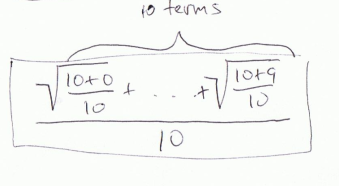As an example, if all of the ten terms on the top were a "1" - for example, if it were like this: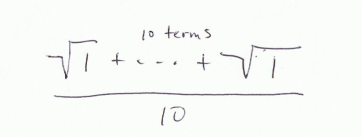You should be able to easily see that it totals up to 1... Slicing n ones into pieces of 1/nth is like adding up n ones and dividing by n... which adds up to one.

Now, going back to the original problem, I'll write out the expansion of the summation in terms of n... so for the first term, we replace k with 0, and for the last, we replace k with (n-1).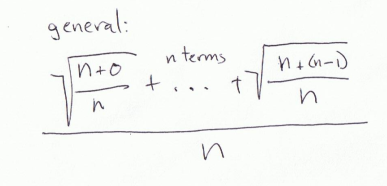What happens as n approaches infinity? Ignore the bottom n for now; maybe you see why I pulled it down there now, because if you leave it in front of each term it can confuse.

The first term: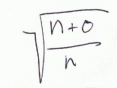Will always be 1 inside the radical. And the square root of one is one. So the first term will always be one.

The last term: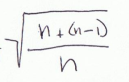Will have the numerator get closer and closer to double the denominator. As n goes to infinity, it will approach 2n/n... which is 2. So the last term becomes the square root of 2.

So the general, again: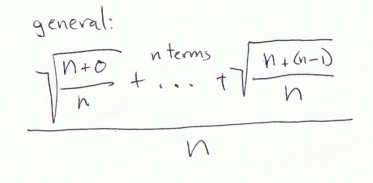when n goes to infinity, will reduce to: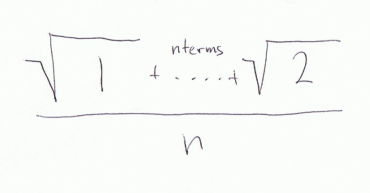So you're covering the range from radical 1 to radical 2... but you're dividing it into thin slices. It's kinda like finding the average value between 1 and radical 2 - whihc woudl be (1 + 1.4142135 ) / 2, or 1.207107... which makes intuitive sense. It's a little bit more than one... when all the terms on top were a 1, add up ten million of them and divide by ten million, you get a 1. Instead, you're adding up ten million terms which go from 1 to 1.4142135, and then dividing by ten million. Makes sense? But it's not quite right...

Because that would only work if the numbers between radical 1 and radical 2 progressed linearly. They don't, because the radical function makes a curve, not a line. Going back to my terrible drawing:That's the curve of the radical funcion f(x) = SQRT(x). (The line should get up to 2 when x is 4, and should get up to 3 when x is 9.)

But for us, only a small region matters.... at the beginning of the expansion, when k is zero, the term simplifies to 1. And at the end, where k gets as big as n, it approaches 2. So that's the range I want to shade in, from 1 to 2. What's the top bound? The radical x function... so veriticall, I go up to radical (1) at the start, and up to radical (2) at the end.

Satisfyingly, this area is going to be a hair larger than my initial estimate of 1.207... I drew a horizontal line at y = 1 for convenience; the shaded area below that has an area of 1. If the shade area extended up to the dotted line at 1.414, the area would be 1.414. If the curve from (1,1) to (2,1.414) were a straight line, then 1.207 would be correct - but it's not a straight line, it's a curve the bellies up. So the real answer is just over 1.207.

At this point, I could easily do the integral of the radical x function from 1 to 2 to get the exact numerical answer... but I'm certain that this is NOT the approach the calc 2 prof would want to see. Even if I'm right, I'm getting at the answer the wrong way.##### Full House
I get the answer 4/3 SQRT (2) - 2/3... which is about 1.2189.

I'm pretty sure that's the right answer - similar, but not quite 2/3rds....
4/3 - 2/3 = 2/3 = .666666...

But I think it's

4/3 SQRT(2) - 2/3 = 1.2189...

I finally got it, but not on my first or second try. I haven't done an integral for close to 25 years...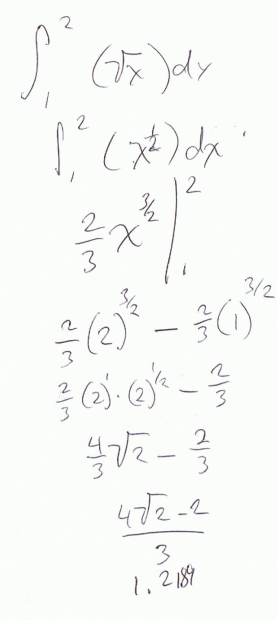but again, I've gotten there the wrong way... so it's quite possibly I've gotten to teh wrong place

##### Royal Flush
Well, for starters, I could just be plain ol' wrong. But here's how I looked at different parts of the construction to try to make intuitive and visual sense out of the exapnsion, and what happen when you approach infinity... I'm going to walk through my logic step-by-step, so you can see how I got there (or top me right where I'm making a goof.)

First, to make sense of the 1/n part in the front, which can easily confuddle - your expansion will always have n terms to sum... and each of them will be multiplied by 1/n. So, for example, if n is ten, you have ten terms, each multiplied by 1/10th. That means this:

View attachment 11149

It's like slicing the series into ten pieces and then adding them up. I can make better sense of what I'm "slicing up" if I move the 1/10th... like so:

View attachment 11150

As an example, if all of the ten terms on the top were a "1" - for example, if it were like this:

View attachment 11151

You should be able to easily see that it totals up to 1... Slicing n ones into pieces of 1/nth is like adding up n ones and dividing by n... which adds up to one.

Now, going back to the original problem, I'll write out the expansion of the summation in terms of n... so for the first term, we replace k with 0, and for the last, we replace k with (n-1).

View attachment 11157

What happens as n approaches infinity? Ignore the bottom n for now; maybe you see why I pulled it down there now, because if you leave it in front of each term it can confuse.

The first term:
View attachment 11158

Will always be 1 inside the radical. And the square root of one is one. So the first term will always be one.

The last term:
View attachment 11159

Will have the numerator get closer and closer to double the denominator. As n goes to infinity, it will approach 2n/n... which is 2. So the last term becomes the square root of 2.

So the general, again:

View attachment 11160

when n goes to infinity, will reduce to:

View attachment 11161

So you're covering the range from radical 1 to radical 2... but you're dividing it into thin slices. It's kinda like finding the average value between 1 and radical 2 - whihc woudl be (1 + 1.4142135 ) / 2, or 1.207107... which makes intuitive sense. It's a little bit more than one... when all the terms on top were a 1, add up ten million of them and divide by ten million, you get a 1. Instead, you're adding up ten million terms which go from 1 to 1.4142135, and then dividing by ten million. Makes sense? But it's not quite right...

Because that would only work if the numbers between radical 1 and radical 2 progressed linearly. They don't, because the radical function makes a curve, not a line. Going back to my terrible drawing:That's the curve of the radical funcion f(x) = SQRT(x). (The line should get up to 2 when x is 4, and should get up to 3 when x is 9.)

But for us, only a small region matters.... at the beginning of the expansion, when k is zero, the term simplifies to 1. And at the end, where k gets as big as n, it approaches 2. So that's the range I want to shade in, from 1 to 2. What's the top bound? The radical x function... so veriticall, I go up to radical (1) at the start, and up to radical (2) at the end.

Satisfyingly, this area is going to be a hair larger than my initial estimate of 1.207... I drew a horizontal line at y = 1 for convenience; the shaded area below that has an area of 1. If the shade area extended up to the dotted line at 1.414, the area would be 1.414. If the curve from (1,1) to (2,1.414) were a straight line, then 1.207 would be correct - but it's not a straight line, it's a curve the bellies up. So the real answer is just over 1.207.

At this point, I could easily do the integral of the radical x function from 1 to 2 to get the exact numerical answer... but I'm certain that this is NOT the approach the calc 2 prof would want to see. Even if I'm right, I'm getting at the answer the wrong way.Alright, That makes a lot of sense, thanks.

•#### spikeithard

##### Flush
wow I feel pretty stupid now. I can barely do simple math and you guys are doing stuff that looks Japanese to me

•SixSpeedFury

#### spikeithard

##### Flush
OK ... so I know its brackets then exponents... then divide..... somehow I dont think BEDMAS applies here so I am bowing out heheh
I tried

#### abby99

##### Full House
Supporting Member
Thanks for the walk down memory lane. It's been at least 35 years since I took a calculus class and have forgotten most of it, yet I understood Mental Nomad's solutions.•##### Royal Flush
Nomad, thanks for helping my boy out. Much appreciated

•Replies
211
Views
5K
Replies
67
Views
2K
Replies
52
Views
1K
Replies
52
Views
2K
Replies
20
Views
414